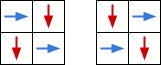시간 제한 메모리 제한 제출 정답 맞은 사람 정답 비율
1 초 256 MB 2 1 1 100.000%

## 문제

There is an $H \times W$ grid. Let $(i,\ j)$ be the cell at the intersection of the $i$-th row ($0 \leq i \leq H-1$) and the $j$-th column ($0 \leq j \leq W-1$). Initially, there is an eel at the cell $(0,\ 0)$. The eel repeats the following process.

• If the current cell is painted, end the process.
• If the current cell is not painted, paint the cell and move to another cell. If the current cell is $(i,\ j)$, the new cell must be either $((i+1)\ {\rm mod}\ H,\ j)$ or $(i,\ (j+1)\ {\rm mod}\ W)$.

Count the number of ways to paint all cells and end the process at the cell $(0,\ 0)$, modulo $10^9+7$. Two ways are considered distinct if the path traveled by the eel are distinct.

## 입력

$H$ $W$

## 출력

Print the answer modulo $10^9+7$.

## 제한

• $2 \leq H, W \leq 10^6$

## 예제 입력 1

2 2


## 예제 출력 1

2


## 예제 입력 2

6 3


## 예제 출력 2

3


## 예제 입력 3

3 4


## 예제 출력 3

0


## 예제 입력 4

10 10


## 예제 출력 4

260


## 예제 입력 5

200 300


## 예제 출력 5

551887980


## 힌트

The following picture shows the two ways in Sample 1: